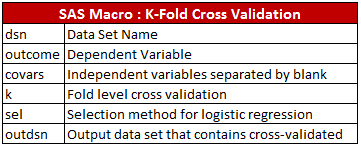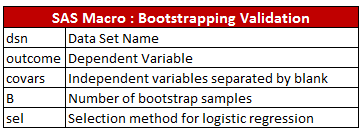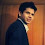# Model Validation Techniques

This articles discusses about various model validation techniques of a classification or logistic regression model. The below validation techniques do not restrict to logistic regression only. It can be used for other classification techniques such as decision tree, random forest, gradient boosting and other machine learning techniques. These validation techniques are considered as benchmarks for comparing predictive models in marketing analytics and credit risk modeling domain. Model validation is a crucial step of a predictive modeling project.

Primarily there are three methods of validation. They are listed below -

1. Split Sample Validation
2. Cross Validation
3. Bootstrapping Validation

The detailed explanation of these methods are listed below -

1. Split Sample Validation
1. Randomly split data into two samples: 70% = training sample, 30% = validation sample.
2. Score (predicted probability) the validation sample using the response model under consideration.
3. Rank the scored file, in descending order by estimated probability
4. Split the ranked file into 10 sections (deciles)
5. Number of observations in each decile
6. Number of actual events in each decile
7. Number of cumulative actual events in each decile
8. Percentage of cumulative actual events in each decile. It is called Gain Score.
9. Divide the gain score by % of data used in each portion of 10 bins. For example, in second decile, divide gain score by 20.
10. Calculate KS statistics (It measure of the degree of separation between the positive and negative distributions. In other words, it checks the maximum difference between distribution of cumulative events and cumulative non-events)

Model Validation Metrics

1. KS Statistics

KS Test measures to check whether model is able to separate events and non-events. In probability of default (bank defaulters) model, it checks whether the credit risk model is able to distinguish between good and bad customers. The calculation of KS test is explained below -
KS = Maximum difference between Cumulative % Event and Cumulative % Non-EventKS Test

Important Note - In this case, KS is maximum at third decile and KS score is 59.1. Ideally, it should be in first three deciles and score lies between 40 and 70. And there should not be more than 10 points (in absolute) difference between training and validation KS score. Score above 70 is susceptible and might be overfitting so rigorous validation is required.

Calculating KS Test with SAS

2. Rank Ordering

To see rank ordering, calculate the percentage of events (defaults) in each decile group and check the event rate should be monotonically decreasing. It means the model predicts the highest number of events in the first decile and then goes progressively down. You can check the rank ordering in the image below. The rank ordering is maintained in this example. It is a simple line graph of percentage of events against deciles (scoring bins).Rank Ordering

3. Area under curve

It explains the trade-off between true positive rate (Sensitivity) and false positive rate (1-Specificity). It is calculated by summing Concordance value and (0.5 times of Tied Percent).ROC Curve
AUC should be more than 0.7 in both the training and validation samples. Should not be a significant difference between AUC score of both these samples. If it is more than 0.8, it is considered as an excellent score.

4. Hosmer Lemeshow Test

It measures calibration and shows how close the predicted probabilities are to the actual rate of events. The p-value should be greater than 0.05, it means model fits data well. This rule might be tough to achieve if you are working on large sample and small event rate.

5. Lift Chart

It measures how much better one can expect to do with the predictive model comparing without a model.
Understand Gain and Lift Chart

Model Validation Rules : Summary
1. Same significant variables should come in both the training and validation sample.
2. The behavior of variables should be same in both the samples (same sign of coefficients)
3. Beta coefficients should be close in training and validation samples
4. KS statistics should be in top 3 deciles.
5. KS statistics should be between 40 and 70. Should not be significantly different from Training KS (more than 10 points in absolute)
6. Rank Ordering - There should not be any break in rank ordering.
7. Lift Curve - The larger the cumulative lift value the better the accuracy
8. Area under Curve (AUC) - Should be more than 0.7.
9. Goodness of Fit Tests - Model should fit the data well. Check Hosmer and Lemeshow Test and Deviance and Residual Test.
SAS Code : Model Validation - Logistic Regression
/* Split data into two datasets : 70%- training 30%- validation */
Proc Surveyselect data=finaldata out=split samprate=.7 outall;
Run;

Data training validation;
Set split;
if selected = 1 then output training;
else output validation;
Run;
/* Logistic Model*/
ods graphics on;
Proc Logistic Data = training;
Model Sbp_flag = age_flag sex bmi_flag/ lackfit ctable pprob =0.5 outroc=troc;
Output out= test p=ppred;
score data=validation out = Logit_File outroc=vroc;
Run;

Proc rank data= logit_file descending groups=10 out=predrank_Dev;
var P_1 ;
/****PREDRANK - variable name for ranks****/
ranks predrank;
run ;
PROC TABULATE Data= predrank_Dev;
CLASS predrank;
VAR Sbp_flag;
TABLE predrank=" " all, Sbp_flag*(N="Count" SUM="Number of Responses" COLPCTSUM="% of events") / box="Decile";
TITLE Creating a Lift Table for Model;
RUN;

Proc Freq data = predrank_Dev;
tables Sbp_flag;
run;
Proc npar1way data=logit_file edf;
class Sbp_flag;
var p_1;
run;

2. Cross Validation

1. Jack-knife / Leave-one-out : The model is fitted on all the cases except one observation and is then tested on the set-aside case. This procedure can be repeated as many times as the number of observations in the original sample (random without replacement sampling). It is implemented in PROC LOGISTIC with predprobs=crossvalidate.

Limitation :

If the model is tested on a single observation, it is not possible to assess one of the most important dimensions of model’s performance, i.e. calibration (measure of how close the predicted probabilities are to the actual rate of events).

2. K-fold cross-validation : Splits the data into K subsets; each is held out in turn as the validation set. (Random without replacement technique)

10-fold cross- validation :
1. Randomly divide your data into ten parts.
2. Hold aside the first tenth of the data as a validation dataset; fit a logistic model using the remaining 9/10 (the training dataset).
3. Using the resulting training model, calculate the predicted probability for each validation observation.
4. Repeat this 9 more times (so that each tenth of the dataset becomes the validation dataset exactly once).
5. Now, you have a predicted probability for each observation from a model that was not based on that observation.
6.  An AUC score is calculated for each of the 10 runs, and then calculate average AUC.
SAS Code : Jack-knife / Leave-one-out Validation

proc logistic data=fil.liver descending;
model complications = age_at_op comorb lobeormore_code bilat_resec_code numsegs_resec;
output out=preds predprobs=crossvalidate;
run;

ods select none;
ods output WilcoxonScores=WilcoxonScore;
proc npar1way wilcoxon data= preds;
where complications^=.;
class complications;
var  XP_1;
run;
ods select all;

data AUC;
set WilcoxonScore end=eof;
retain v1 v2 1;
if _n_=1 then v1=abs(ExpectedSum - SumOfScores);
v2=N*v2;
if eof then do;
d=v1/v2;
Gini=d * 2;
AUC= d + 0.5;
put AUC=  GINI=;
keep AUC Gini;
output;
end;
run;

proc logistic data=preds descending;
model complications = age_at_op comorb lobeormore_code bilat_resec_code numsegs_resec;
roc pred=xp_1;
roccontrast;
run;

Rule : Compare area under curve of both the samples.

SAS Macro : K-Fold Cross ValidationSAS Macro : K-Fold Cross Validation
The following SAS program was written by Mithat Gonen. I modified it to calculate AUC of validation dataset and store modeling results of each fold in a dataset.

%macro xval(dsn=,outcome=,covars=,k=10,sel=stepwise,outdsn=_xval_,outdsn2=comparison);

data _modif;
set &dsn;
unif=&k*ranuni(20052905);
xv=ceil(unif);
run;

%do i=1 %to &k;
proc logistic data=_modif(where=(xv ne &i)) outmodel=_mod&i;
model &outcome (event="1") =&covars / selection=&sel;
ods output association=assoc&i;
run;
%if print^=0 %then %do;proc printto file='junk.txt';%end;
proc logistic inmodel=_mod&i;
score data=_modif(where=(xv=&i)) out=out&i;
run;

ods select none;
ods output KolSmir2Stats=KS&i;
proc npar1way data= out&i edf;
where &outcome^=.;
class &outcome;
var  P_1;
run;
ods select all;

ods select none;
ods output WilcoxonScores=Wil&i;
proc npar1way wilcoxon data= out&i;
where &outcome^=.;
class &outcome;
var  P_1;
run;
ods select all;

data AUC&i;
set Wil&i end=eof;
retain v1 v2 1;
if _n_=1 then v1=abs(ExpectedSum - SumOfScores);
v2=N*v2;
if eof then do;
d=v1/v2;
/*Gini=d * 2; */
Scoring_AUC= d + 0.5;
put Scoring_AUC=;
put "****Open work.results dataset to see results of training datasets....";
keep Scoring_AUC;
output;
end;
run;

%if print^=0 %then %do;proc printto;run;%end;
%end;
data &outdsn;
set %do j=1 %to &k;out&j %end;;
run;

data training (keep =label2 nvalue2 rename= (nvalue2=Training_AUC));
set %do j=1 %to &k;assoc&j %end;;
where label2= 'c';
if label2='c' then label2 ='AUC';
run;

data ks (keep =label2 nvalue2 rename= (nvalue2=Scoring_KS));
set %do j=1 %to &k;ks&j %end;;
where label2= 'D';
if label2='D' then label2 ='KS';
run;

data validation;
set %do j=1 %to &k;AUC&j %end;;
run;

data &outdsn2 (drop = label2);
merge training validation ks;
run;

ods select none;
ods output WilcoxonScores=WilcoxonScore;
proc npar1way wilcoxon data= &outdsn;
where &outcome^=.;
class &outcome;
var  P_1;
run;
ods select all;

data AUC;
set WilcoxonScore end=eof;
retain v1 v2 1;
if _n_=1 then v1=abs(ExpectedSum - SumOfScores);
v2=N*v2;
if eof then do;
d=v1/v2;
Gini=d * 2;
AUC= d + 0.5;
put AUC=  GINI=;
put "****Open work.results dataset to see results of training datasets....";
keep AUC Gini;
output;
end;
run;

%mend;

%xval(dsn=alldata,outcome=y,covars=entry,k=5,sel=stepwise, outdsn=kfold, outdsn2=comparison);

Note: Calculate AUC of each of the runs and then calculate average of all of the 10 runs.

3. Bootstrapping Validation

The bootstrap method first generates N bootstrap samples (sample with replacement) drawn from the original sample. On each of these bootstrap samples the model is estimated and the performance was measured both on the bootstrap sample and on the original sample. The average difference between the two performance measures forms an estimate of the optimism.

In other words, you randomly draw your observations with replacement, then calculates the logistic regression and stores the coefficients. This is repeated n times. So you'll end up with 10'000 different regression coefficients.

Rule : If a variable is truly representative of the model it will occur in the majority of the N fitted models. The c statistics is calculated for each iteration in order to examine the predicted probability of each model.The overall accuracy is the average of the N measures.SAS Macro : Bootstrapping Validation
The following SAS program was written by Mithat Gonen.

%macro bval(dsn=,outcome=,covars=,B=10,sel=stepwise);

proc sql noprint;
select n(&outcome) into:_n from &dsn;
run;

proc surveyselect data=&dsn method=urs outhits rep=&B n=&_n out=bsamples noprint;
run;

%do i=1 %to &B;
proc logistic data=bsamples(where=(replicate=&i)) outmodel=_mod&i descending noprint;
model &outcome=&covars / selection=&sel;
run;

proc printto file='junk.txt';
proc logistic inmodel=_mod&i;
score data=&dsn out=out1&i;
run;

proc logistic inmodel=_mod&i;
score data=bsamples(where=(replicate=&i)) out=out2&i;
run;
proc printto;run;
%end;
data bval1;
set %do j=1 %to &B;out1&j(in=in&j) %end;;
%do j=1 %to &B; if in&j then bsamp=&j; %end;
run;
data bval2;
set %do j=1 %to &B;out2&j(in=in&j) %end;;
%do j=1 %to &B; if in&j then bsamp=&j; %end;
run;

proc printto file='junk.txt' new;
proc logistic data=bval1 descending;
by bsamp;
model &outcome=p_1;
ods output association=assoc1;
run;

proc logistic data=bval2;
by bsamp;
model &outcome=p_1;
ods output association=assoc2;
run;
proc logistic data=&dsn;
model &outcome=&covars / selection=&sel;
ods output association=assoc3;
run;
proc printto;

data assoc3;
set assoc3;
bsamp=1;
run;

data optim;
merge assoc1(where=(label2='c') keep=bsamp label2 nvalue2 rename=(nvalue2=auc1))
assoc2(where=(label2='c') keep=bsamp label2 nvalue2 rename=(nvalue2=auc2))
assoc3(where=(label2='c') keep=bsamp label2 nvalue2 rename=(nvalue2=auc3));
by bsamp;
run;

proc sql;
select mean(auc3) as OptimisticAUC, mean(auc2-auc1) as OptimisimCorrection,
mean(auc3)-mean(auc2-auc1) as CorrectedAUC from optim;
quit;
%mend;

%bval(dsn=liver,outcome=complications,covars=age_at_op comorb lobeormore_code bilat_resec_code numsegs_resec,B=10);
Related Posts
ShareDeepanshu founded ListenData with a simple objective - Make analytics easy to understand and follow. He has over 10 years of experience in data science. During his tenure, he worked with global clients in various domains like Banking, Insurance, Private Equity, Telecom and HR.

14 Responses to "Model Validation Techniques"
1.Thanks mate for all your hardwork.

Ks statistics using npar1way is recorded in decimals(fragments) with p-value. How do u get 40-70?

After getting the ranked probabilities, how do u know wch customer belongs to a decile?

2.Thanks mate for all your hardwork.

Ks statistics using npar1way is recorded in decimals(fragments) with p-value. How do u get 40-70?

After getting the ranked probabilities, how do u know wch customer belongs to a decile?

1.By 40-70, i meant 40% - 70% (0.4 - 0.7). Run PROC RANK with GROUPS = 10 to know the customer placement in a decile.

3.Nice work, Deepanshu! The way you listed steps and SAS codes for model validation in logistic regression is really helpful. It would be more helpful if you have a one line statement regarding each SAS code stating what it is doing and where does it belong in the 10 steps split sample validation.

Thanks,
VB

4.The bootstrap validation code seems to produce an error message. The statement below

proc logistic data=bval1 descending;
by bsamp;
model &outcome=p_1;
ods output association=assoc1;
run;

is where the problem is -- SAS complains that the "Variable "p_1 is not found". The same error occurs in PROC LOGISTIC for the data=BVAL2.

5.6.Really helpful post Deepanshu, I wanted to know the thoery behind calculation of KS statistic, Gini Coefficient. Also how to calculate the GOF value?

7.Its good work

8.awesome job Deepanshu,

But on the theoretical note, how to we score with bootstrapping logistic regression ??

9.Pardon,
where can I find the dataset of the previous SAS codes?

10.Will you please do the same (or provide code) with the GLM model.

11.What if I have breaks in rank ordering ? How to remove those breaks

12.Hi Deepanshu,

What if we get KS statistic in 4th decile.

Regards,
Harneet.

13.I am getting crack(break) in rank ordering need....

Next → ← Prev
Looks like you are using an ad blocker!

To continue reading you need to turnoff adblocker and refresh the page. We rely on advertising to help fund our site. Please whitelist us if you enjoy our content.